HomeArticlesProjectsBlogContact
Articles
How A Diode WorksColin Mitchell
May 25, 2021
Make sure to subscribe to our newsletter and be the first to know the news.

01
WHICH WAY DOES CURRENT FLOW?
02
WE WILL TAKE THE EXAMPLE OF A SILICON DIODE
03
DIODE VOLTAGE NOT CONSTANT
04
REVERSE VOLTAGE
05
DIODE PUZZLE
06
DIODE APPLICATIONS
07
THE DIODE AS A PROTECTION DIODE
08
THE DIODE CONVERTS AC TO DC
09
FULL WAVE RECTIFICATION
10
What happens when a diode goes "OPEN?"
11
What happens when a diode goes "SHORT-CIRCUIT?"
12
POSITIVE AND NEGATIVE STUD MOUNT DIODES
13
TESTING A STUD MOUNT DIODE
14
THE HEATSINK
15
THE ZENER DIODE
16
THE ZENER ANALOGY
17
THE TRANSISTOR AS A ZENER DIODE
18
THE SHUNT REGULATOR
19
ZENER QUESTION
20
THE SERIES REGULATOR
21
CURRENT SHARING
22
ACTUAL ZENER CIRCUIT
23
GATING - how a diode can be used as a GATE
24
GATING AN OSCILLATOR
25
THE GATING DIODE
26
DIODE GATES
27
THE DIODE OR GATE
28
THE DIODE AND GATE
29
THE TRANSISTOR
30
BREAKDOWN
31
WHY DOES A DIODE FAIL?
32
HEATSINKING
33

#### (a Self-teaching Guide)

DIODE TEST

More on the diode in: Basic Electronics 1A

There is a lot of confusion in text books and on the web about CURRENT FLOW.

## .css-1qh5hbx{box-sizing:border-box;margin:0;min-width:0;display:block;color:var(--theme-ui-colors-heading,#2d3748);font-weight:bold;-webkit-text-decoration:none;text-decoration:none;margin-bottom:1rem;font-size:1.5rem;position:relative;}WHICH WAY DOES CURRENT FLOW?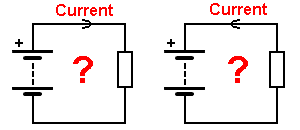Current is a flow of electrons. These electrons are negatively charged particles and they are attracted to the POSITIVE of the supply. This means they flow from NEGATIVE to POSITIVE.
The first inventors and discoverers of electricity did not know this.
They thought electricity flowed from POSITIVE to NEGATIVE.
So, they made a CONVENTION (statement) that electricity (CURRENT) flows from POSITIVE to NEGATIVE.

They were wrong. But hundreds of text books had already been written, so we have TWO situations.

When we discuss electrical and electronic circuits, we use the old convention, called CONVENTIONAL CURRENT FLOW (from positive to negative). To get away from any idea of thinking about “electrons” we say ”electricity flows from positive to negative.” We say this so we can follow all the electrical and electronic circuits using arrows that point in the direction of CONVENTIONAL CURRENT FLOW.

When discussing ELECTRON-FLOW we use NEGATIVE to POSITIVE.
We keep ELECTRON FLOW arrows within the component we are talking about (such as a radio-valve or transistor-model) and do not put electron-flow arrows on the rest of the circuit.
We have to do this to prevent CONFUSION.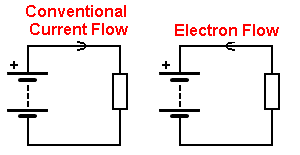The Electron Flow arrow should be within the component and NOT on the wiring

Don’t use any text-books that say current flow is electron-flow as they are omitting CONVENTIONAL CURRENT FLOW and this will confuse you.

We are discussing this point because a diode is an ELECTRONIC device. In other words it involves the flow of electrons because CURRENT will only flow in one direction through a diode.
In all of our discussions we have used CONVENTIONAL CURRENT FLOW as we are talking to beginners in electronics and not PHYSICS students.
Some text books use one convention, then the other convention and nothing can be followed.
Note: the arrow on the emitter of a transistor follows conventional current flow, even though electron flow was known at the time.

A diode is a very simple device and it has a lot of applications. We will cover some of its uses and explain exactly how it works in very simple terms.
If you don’t understand any of the points in this discussion, you can contact Colin Mitchell.

A diode is a device that passes current in only one direction. It is a bit like a water-valve that prevents water back-flowing into the mains from your property. Or a valve in a pump that prevents the water flowing back down a well.

There are many types of diodes to handle small currents, large currents, high frequencies and high voltages. And there are diodes made from different materials, but they can all be described in a simple way. And that’s what we will do.

A diode has two leads. These are called ANODE and CATHODE.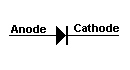The cathode end is identified in a circuit diagram and on the body of the device.
It may be identified with a line, chamfer or dimple or a symbol. There must be something on the diode that identifies this lead and you have to look for it.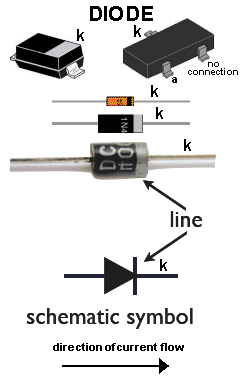A diode does NOT have a positive or negative end. You see this mistake in so many discussions. A diode will have a positive voltage on the anode and a slightly lower (positive) voltage on the cathode. It will not have a positive on the anode and negative on the cathode.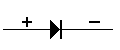Incorrect marking with ”+” and ”-”

In the following diagram only the CATHODE is identified with the letter k (for kathode). The other lead is the ANODE. And that’s the only way to identify a diode. That’s the professional way.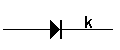Correct marking with “k”

Here is a pictorial way to understand how a diode works: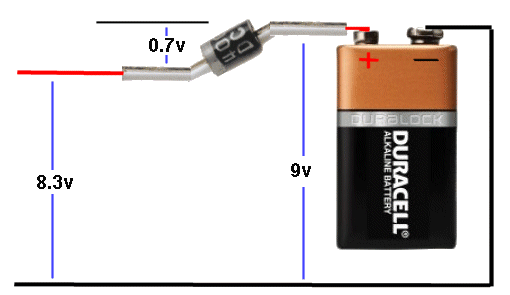OR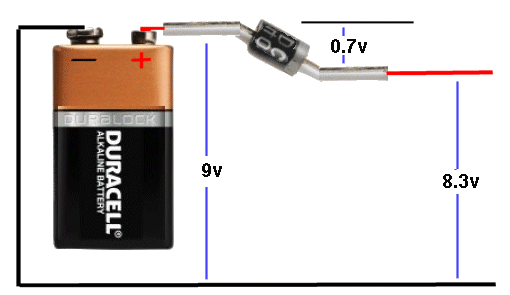IT DOES NOT MATTER WHICH WAY YOU DRAW THE CIRCUIT, THE RESULT IS THE SAME

9v comes out of the battery and when it passes through the diode, 0.7v is LOST (dropped across the diode), resulting in 8.3v available to operate a motor etc. It does not matter if you are driving a motor or a torch globe, the device will see 8.3v But the actual voltage across the device will depend on the current flowing and it will be 8.3v or lower depending on the surface area of the junction of the diode and how much stress you are placing on the diode. If the diode is designed to pass 10 amps and the current flowing is 1 amp, the voltage across it will be 0.7v because it is not being “overloaded.” But 1 amp through a 1amp diode is going to stress it enormously and the voltage across the junction can go as high as 1.1v. So, nothing is predictable and you have to build the circuit to find out.

The most common type of diode is made from SILICON. It can also be made from GERMANIUM. You need to look in the datasheet to find the composition of the diode you are using. 99% of the time it will be silicon.

A diode does not start to TURN ON until a small voltage is present on its ANODE lead.
For a Germanium diode, this voltage is approx 0.3v.
For a Schottkey diode, this voltage is 0.3v
For a Silicon diode, this voltage is 0.7v. When the voltage on the Anode is 0.8v, you will get 0.1v on the Cathode. And the same principle applies with all diodes.

You must NEVER put a diode across the leads of a power supply. It will blow up.
A diode does not determine the amount of current flowing. The current flowing is determined by the load (such as a motor) and the voltage of the battery.
This is a simple OHM’s LAW equation and to get an exact answer, just take 0.7v from the supply voltage and put the values into the equation.

Question For a diode, does current flow from anode to cathode, or cathode to anode?

Answer Current flows from anode to cathode. The arrow on the symbol shows the direction of CONVENTIONAL CURRENT flow.

## WE WILL TAKE THE EXAMPLE OF A SILICON DIODE

A silicon diode is just like the wall of a dam. As soon as the water reaches the top of the wall, it overflows. The silicon diode has a height of 0.7v and as the voltage from the power supply increases, nothing happens until the voltage reaches 0.7v. At this voltage CURRENT STARTS TO FLOW and if the voltage is increased, the CURRENT INCREASES. It’s just like the water flowing over the top of the dam.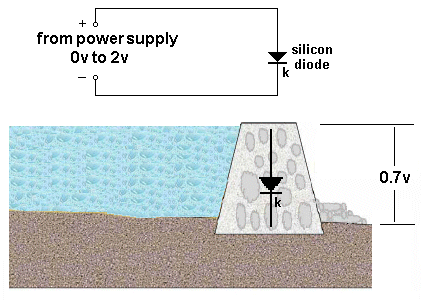## DIODE VOLTAGE NOT CONSTANT

We have said the voltage across a silicon diode is 0.7v. This voltage increases slightly as the current increases.
For a 1 amp diode, this voltage will increase to about 1.1v when 1 amp is flowing.
For a 3 amp diode, this voltage will increase to about 1.1v when 3amp is flowing.
For a 10 amp diode, this voltage will increase to about 1.1v when 10 amp is flowing.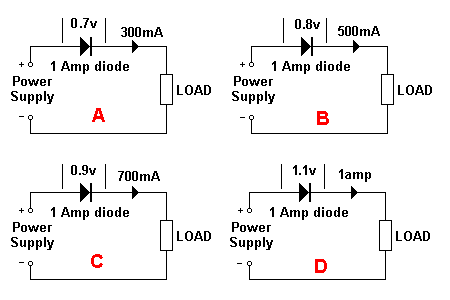The voltage across a diode increases with current-flow

In the diagrams above, the current-flow is determined by the voltage of the Power Supply AND the current required by the load. The diode does NOT determine the current-flow. By increasing the voltage of the Power Supply, the current will increase.
The LOAD can be a motor, globe or high-wattage resistor.
In diagram A, the voltage drop (voltage lost) across the diode is 0.7v. This voltage increases to 1.1v in diagram D due to the increase in current.
Because the current and voltage increases in each diagram, the wattage (heat generated) by the diode increases at a very fast rate:
In diagram A the wattage generated by the diode is: 0.3 x 0.7 = 0.21watts
In diagram B the wattage generated by the diode is: 0.5 x 0.8 = 0.4watts
In diagram C the wattage generated by the diode is: 0.7 x 0.9 = 0.63watts
In diagram D the wattage generated by the diode is: 1.1 x 1 = 1.1watts

## REVERSE VOLTAGE

If you connect a diode around the wrong way, no current will flow. But if you increase the voltage to 100v, 200v or 300v, the diode will suddenly break-down and a current will flow.
The voltage at which this occurs is called the REVERSE BREAKDOWN VOLTAGE.
It could be as low as a few volts or as high as 1,000v.
This voltage is always provided in the data sheet as it is most-important.
If a diode is used in a mains BRIDGE RECTIFIER (to be discussed later), it will see a voltage as high as 325v for the 240v mains and the diode will need to be a 400v device.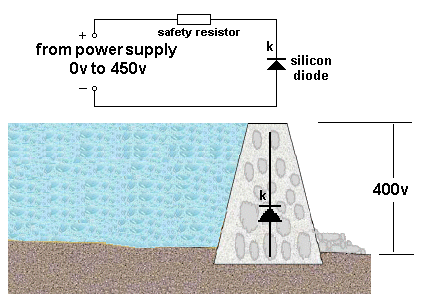A 1N4004 is a 400v diode - This is its REVERSE VOLTAGE rating

In the circuit above, the diode will not be destroyed when the voltage reaches 400v (for a 1N4004) because the current-limiting SAFETY RESISTOR has been included.

Question For a silicon diode, what is the approximate voltage-drop across its leads when it is delivering about 10% of its rated current?

Answer 0.7v This is is the voltage we use for current up to about 40% of maximum current.

Question Which lead of a silicon diode is identified on a circuit diagram?

Question In the following diagram, is the diode conducting?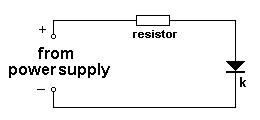Question In the following diagram, is the diode conducting?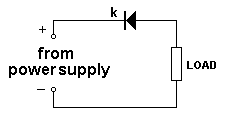Answer No. The diode is reverse-biased. The arrow on the diode indicates the direction of current-flow.

Question In the following diagram, is the diode conducting?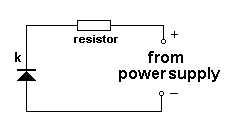Answer No. The diode is reverse-biased. The arrow on the diode indicates the direction of current-flow.

Question In the following diagram, is the diode conducting?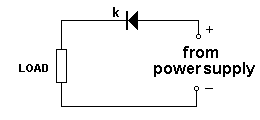## DIODE PUZZLE

The supply in the following two circuits is 12v. Identify the resistors that have current flowing through them: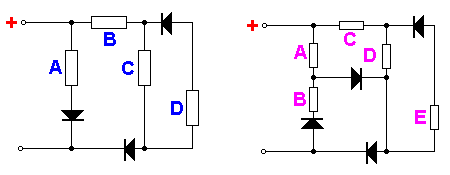(answers are a little further down the page)

## DIODE APPLICATIONS

Here are some applications for a diode (or set of diodes):

## THE DIODE AS A PROTECTION DIODE

In the following diagram, the diode does not conduct if the battery is connected around the wrong way. It is called a PROTECTION DIODE. This means the circuit will not see a reverse voltage and will not be damaged.
However the circuit will see a voltage 0.7v less than the voltage of the battery due to the 0.7v drop (lost) across the diode.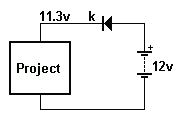Protection diode

In the following circuit, the diode conducts if the battery is connected around the wrong way and creates a SHORT-CIRCUIT. This will burn-out the fuse. The diode is called a PROTECTION DIODE.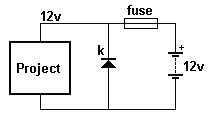Protection diode

The advantage of this circuit is the diode does not drop 0.7v between the supply and the voltage on the project. The full voltage of the supply is connected directly to the project. This is a big advantage for projects working on 12v (battery) and require a high current, however the fuse will be damaged if the battery is connected around the wrong way.

Question In the following diagram, describe the fault: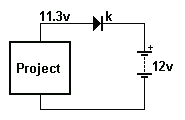Answer The diode has been drawn around the wrong way.

A, B C A C D

## THE DIODE CONVERTS AC TO DC

A diode can be used to convert AC to DC:
The diode converts AC (called ALTERNATING CURRENT) to DC (called DIRECT CURRENT).
Let’s explain this more accurately. Alternating current is the name given to a voltage that rises in value and falls. The electricity supplied to your house is called AC.
One of the wires will have no voltage on it and the other wire will have a voltage that rises to a high value and then reduces to zero and then drops to a high value in the opposite direction.
This is called an ALTERNATING SUPPLY.
If you connect a diode to this wire, the voltage will rise to a high value but when it drops below zero, the diode prevents the negative voltage appearing. The result is this: You only get the positive portion of the voltage and this will be present for half a cycle and nothing will be detected for the half cycle when the voltage is below zero. The result is pulses of positive voltage with a gap between the pulses. We call the result DC but it is really PULSES OF POSITIVE VOLTAGE.
The original household voltage was 120v DC (direct current). DC is the same type of voltage that comes from a battery. It does not rise and fall but is steady at the specified voltage.
If you apply 120v DC to a 120v globe, it illuminates brightly.
You can also operate motors, toasters, heaters and other simple pieces of equipment from DC. (But you cannot operate a piece of equipment containing a transformer and that’s why it has such limited use.)
And you need to locate the generator very close to each household because you cannot use transformers to deliver the supply and there is a voltage drop in the street wiring due to the current flowing in the wiring.
This needed lots of small generating plants and it was a very expensive way to deliver electricity.
The solution was to convert to AC (alternating current) and locate a single generating plant at either a source of coal or water for hydro electricity.
But since AC is rising and falling, the heat produced by 120v AC in a toaster (for example) will not be as much as 120v DC because part of the waveform is less than 120v for a portion of the cycle.
The answer was to make the 120v AC rise higher than 120v for part of the cycle so that it produced the same heat as 120v DC.
This means the 120v AC rises to 170v at its peak.
For 240v AC mains, the voltage rises to 336v.
That’s why the 120v or 240v mains is so dangerous. Your body actually detects the 336v and this is what kills you.
Now, let’s understand how this 120v/240v is delivered.
The mains actually consists of a single wire. This is called the ACTIVE.
The other wire is the GROUND or EARTH or NEUTRAL.
Sometimes the Neutral is also delivered as a wire, but let’s take the case of a single ACTIVE wire.
The voltage in the active wire is rising 336v HIGHER than earth then it falls to 336v LOWER than earth. It is rising and falling like this 50 or 60 times per second.
Once you understand this concept, you will able to see how a diode converts AC to DC.

Placing a diode on the active line of the mains will remove the part of the waveform that falls 336v LOWER than earth.
The result is a set of 50 or 60 pulses per second that rise 336v higher than earth. In actual fact a pulse rises 336v then falls to zero. There is no waveform during the time when the original waveform falls below earth.
The following diagram shows the result of adding a diode to the active line. The result is not DC but PULSATING DC and needs to be smoothed via an electrolytic to get DC.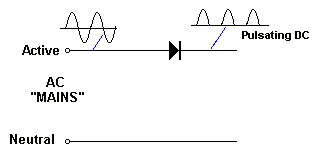The following diagram shows the result of adding an electrolytic::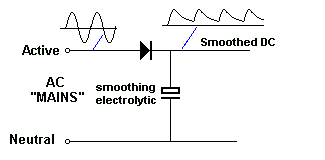Using a single diode is called HALF-WAVE RECTIFICATION.
Adding an electrolytic is called SMOOTHING. It can also be called REDUCING THE RIPPLE.

## FULL WAVE RECTIFICATION

The negative portions of an AC waveform can be combined with the positive portions to produce a pulsing DC waveform.
See: http://www.electronics-tutorials.ws/diode/diode_6.html
or:
http://www.eecs.tufts.edu/~dsculley/tutorial/diodes/diodes3.html
for an explanation on how the diodes in the bridge “guide” the incoming waveform to produce pulsing DC: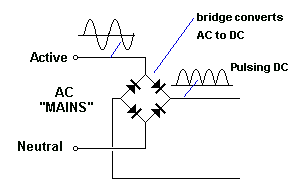When an electrolytic is added to the circuit, it charges during the peaks and delivers energy when the waveform drops. The result is called DC with Ripple.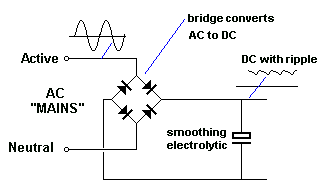If this circuit is not connected to a LOAD, the ripple will be almost zero. If the load takes a small current, the ripple will be small. If the current is large, the ripple will be very large.

## What happens when a diode goes “OPEN?”

The output consists of peaks and spaces: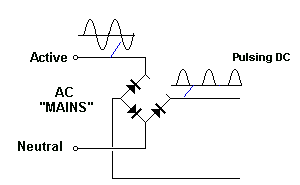When an electrolytic is added, the output voltage looks something like the following, depending on the value of the electrolytic and the current taken by the load. The audio will have a distinct 50Hz hum or “buzz.”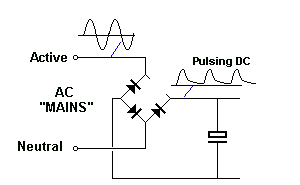What will actually happen is the positive rail will still remain positive but it will be very close to the voltage of the neutral input and the 0v of the supply will be LOWER THAN THE NEUTRAL RAIL.
In other words, the output voltage will “shift down” (relative to the input waveform) but because the output voltage is considered as a “floating voltage” you will not be aware of this.
This fault highlights the dangers in producing a power supply without a transformer.
Normally, the power supply will have the 0v rail connected to components and maybe the chassis or metal case of the project because the 0v rail will be very close to the voltage on the NEUTRAL and this will be very close to “GROUND.” But if a diode goes open, the chassis immediately sees the voltage of the ACTIVE and this can produce an electrical shock.

## What happens when a diode goes “SHORT-CIRCUIT?”

When the active line is positive, the full voltage of the mains appears on the output. But when the mains voltage reverses, the “Neutral” is positive and the voltage passes through Diode D4, DIRECTLY to the active line and this creates a SHORT-CIRCUIT. The current through D4 will be very high and it will be damaged.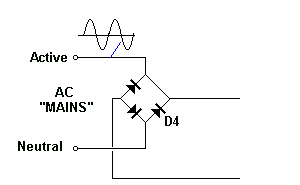Diode D4 will BLOW UP

USING A DIODE AS A VOLTAGE REFERENCE
In the following diagram we see a silicon diode connected to a power supply via a safety resistor: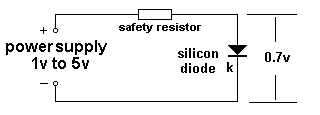The power supply is adjusted from 1v to 5v and the voltage across the diode remains constant at 0.7v.

We can place two or more diodes in series to increase the output voltage: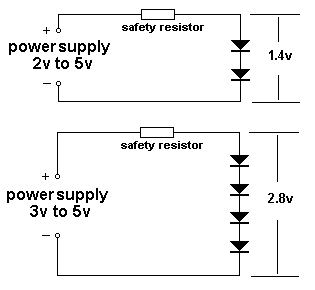In the diagram above you have actually made a 2.8v ZENER DIODE. You can connect an item across the 4 diodes and the circuit will deliver a constant 2.8v, even when the load increases and decreases. This will only occur when the safety resistor has the correct value of resistance and this is covered in our discussion below.

## POSITIVE AND NEGATIVE STUD MOUNT DIODES

There is no such thing as a “Positive or Negative” diode, but in car and truck alternators there are a number of diodes to convert the alternating voltage produced by the alternator to pulsing DC to charge the battery and operate the lighting system.
The battery then becomes a huge storing component to smooth the pulses to get a very smooth DC and that’s why the lights don’t flicker.
The alternator is actually a 3-phase generator as this will produce the maximum output during each revolution.
The three windings are connected to 6 diodes and these diodes are mounted on two plates that act as heatsinks.
Referring to the circuit below you can see the top 3 diodes are connected to the purple plate (heatsink) and the 3 lower diodes to the brown plate (heatsink).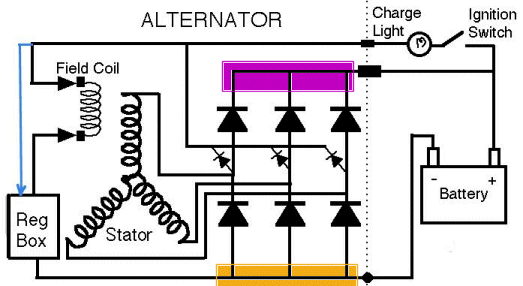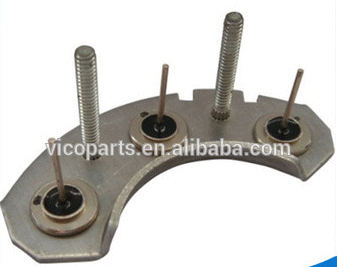The image above shows 3 diodes connected to a plate. They can be ALL positive-mount stud diodes or negative-mount.
Here is a diagram to find positive-mount diodes and negative-mount diodes: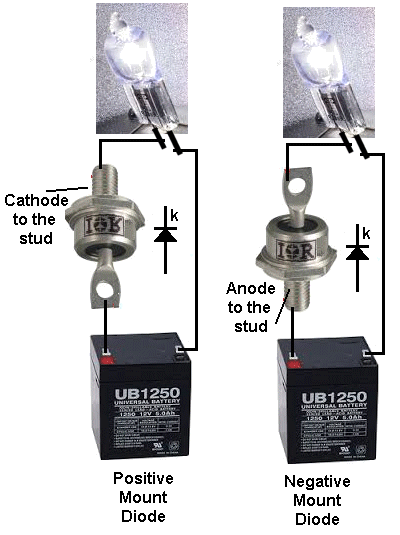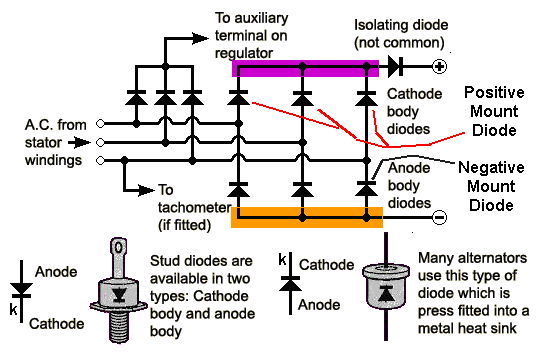This circuit show Positive-mount diodes at the top and Negative-mount diodes at the bottom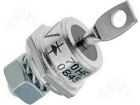Negative Mount Diode Anode Body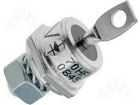Positive Mount Diode Cathode Body

You will notice that both diodes have the same part number and most stud diodes come in either “Stud Cathode” or “Stud Anode.”
You need to make sure which version you are ordering BEFORE placing an order.
Some stud diodes have different colour potting (surrounding the lead) as shown in the following image: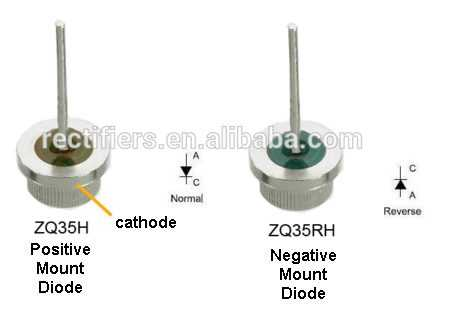A Positive Mount Stud Diode has the cathode connected to the STUD or “body” or screw-thread. This is called a “normal” diode. This is not “electronic” terminology but only refers to stud diodes for alternators.
A reverse diode sometimes has “R” added to the numbering on the component.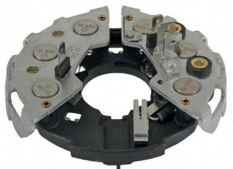The two heatsinks are insulated (isolated) from each other because one is connected to the positive of the battery and the other to the negative of the battery.

## TESTING A STUD MOUNT DIODE

If you have no testing equipment, you can test a diode by connecting a car globe to 2 leads and connecting them to a battery. The globe will illuminate.
Now connect one of the leads to the battery via the diode. The globe will illuminate. Reverse the diode. The globe will not illuminate.
If it illuminates in both directions the diode is faulty (shorted). If the globe never illuminates, the diode is faulty (open).
You can do this will all the diodes on the heatsinks and compare a new diode with the others to make sure you are fitting the correct type of diode.
You MUST do this with every diode before you fit it as this is the only certain way to fit the correct diode. It is so easy to make a mistake.
You can use a multimeter but a globe will not allow a mistake to be made. You can “see” it working.

## THE HEATSINK

The diodes must be mounted on a heatsink because each diode produces a voltage across it of about 0.7v to nearly 1v and when 10 to 20 amps is flowing each diode can produce heat equal to 10 watts. Adding up all the heat being lost can come to 60 watts and this is why the heatsinks get hot and why some of the diodes fail.
Each diode must be firmly attached to the heatsink to allow the heat produced by the diode to be passed to the heatsink.
Diodes cannot be operated “in the air” as they will get very hot and can fail due to overheating.
The only way to test the output of each diode is with a car globe.
You can use up to 100 watts as this will test each diode under load and identify a diode that tests ok with test equipment but fails when a high current flows.

## THE ZENER DIODE

Zener diodes come in voltages from 3v3 to more than 47v. You can consider them to be ordinary diodes that have “failed.” For instance a 1N4001 diode has a reverse breakdown voltage of 50v. It is a 50v ZENER DIODE. A 1N4004 diode has a reverse breakdown voltage of 400v. It is a 400v ZENER DIODE.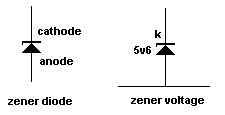Zener Symbol and voltage

A zener diode should always be identified in a circuit with its voltage next to the symbol. You can include the wattage but this can be worked out by looking at the voltage and current flowing though the zener. Don’t use part-numbers to identify the zener voltage as the reader will have to look-up a product-list to find the zener voltage. Circuit diagrams should be instantly readable and instantly understandable.

A zener diode drops 0.7v when connected around one way and drops its ZENER VOLTAGE when connected around the other way. Here is a diagram to show this: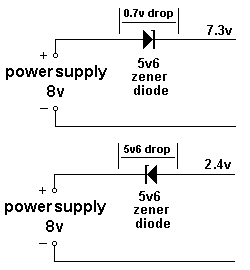The following diagram shows a zener diode producing a constant 5v6 output when the power supply ranges from 6v to more than 8v.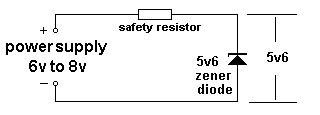If the power supply is adjusted from 0v to 8v, no current flows in the circuit when the voltage is below 5v6. As soon as the power supply reaches 5v6, current flows through the safety resistor and the diode. That’s because the zener diode “breaks down” and current flows through it while at the same time the voltage across the anode and cathode leads is 5.6v
If the power supply voltage is increased, more current flows through the safety resistor and this current also flow through the zener diode. The voltage across the leads of the zener diode remains a constant 5v6. All the increased voltage appears across the resistor.
If the voltage of the power supply is increased further, the current through the safety resistor and the zener diode will increase. Both will get hotter and eventually the zener diode will burn out.

You can produce any zener reference voltage by combining zener diodes and ordinary diodes: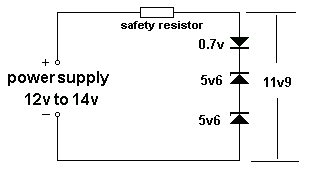Notes:
You can see the two 5v6 zener diodes in the diagram above are connected around the opposite way to the ordinary diode.

Question In the following diagram, what is the combined zener voltage?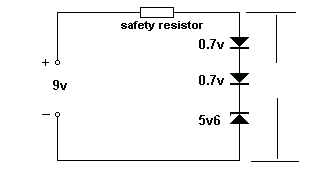Question In the following diagram, what is the combined zener voltage?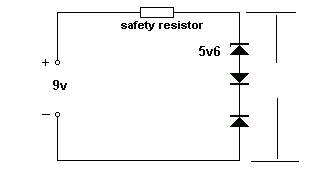Answer One diode is around the wrong way. The circuit will not produce a zener reference voltage. The output voltage will be 9v and the string of diodes will have not effect on determining the output voltage.

Question In the following diagram, If the safety resistor is removed and the 9v supply is connected directly to the two diodes and zener, explain what will happen:Answer A very high current will flow through the diodes and zener because the combined zener of the three items is 7v. They will be damaged.

Question In the following diagram, explain what will happen: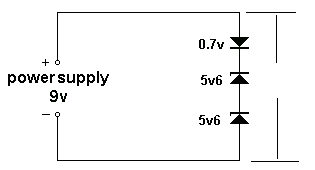Answer The zener value of the three components is 11.9v This voltage is higher than the supply (9v) and NO CURRENT will flow in the circuit.

Question Here’s the most absurd explanation of “Voltage Flow” in a zener diode. Can you see the mistake?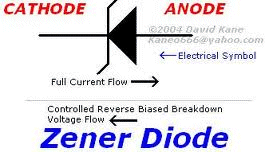Answer Voltage does not “flow.” Voltage is a potential. It “exists” at each point in a circuit. When the voltage on the cathode is higher than the anode, the zener diode will break-down and current will flow from the cathode to anode. When this happens the zener diode is given a number to represent its voltage (break-down voltage).
When the voltage on the anode is higher than the cathode, current flows and the diode acts just like an ordinary diode (none of the zener properties are used) and the only property you need to remember is the current capability of the diode.

Question Zener diodes can be connected in series to produce any voltage. Simply add the zener voltages to provide the resulting output voltage. What is the output voltage of this combination: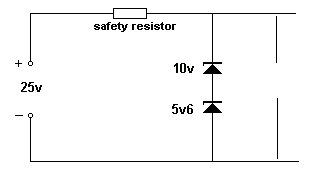Question Insert zener diode A or B to produce an output voltage of 19.4v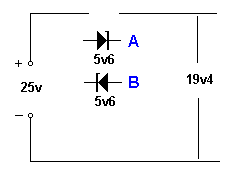Question from a reader: What is the maximum supply voltage for a zener diode?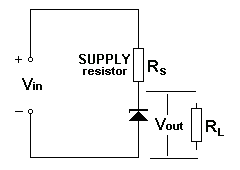Answer Here is the way to work out the Max voltage for Vin:

1. You MUST include a resistor as shown in the diagram above. The resistance of this resistor will be worked out from the following:
2. Select a zener diode for the required output voltage. In other words the ZENER VOLTAGE.
3. Select a 400mW diode, 1watt diode or higher wattage, depending on the current you will be needing.
4. Work out the maximum current though the zener diode:
zener voltage:400mW1 watt10 watt
3v3120mA300mA3 amp
5v175mA200mA2 amp
10v40mA100mA1 amp
12v30mA80mA800mA
15v25mA65mA650mA
24v15mA40mA400mA

MAXIMUM CURRENT THROUGH ZENER
according to the wattage of the device

5. The circuit being designed is called a ZENER REGULATOR or ZENER SUPPLY and the current selected from the table above will flow AT ALL TIMES through the resistor Rs (called the Supply Resistor or safety resistor).
When your load is not taking any current - such as when it is “off” or removed from the circuit, ALL the current will flow through the zener. When you are taking the maximum current, the zener will be taking NO CURRENT. In other words, the two items CURRENT SHARE. They share the maximum current and the sharing can be 0% -100% or 100% - 0%. or any percentage in between.
6. We can now work out the maximum Vin.
7. Vin should be at least 2v higher than the zener voltage. ANY voltage higher than the zener.
8. This voltage is called the HEAD VOLTAGE and any voltage higher than 2v more than the zener voltage is called WASTED VOLTAGE because it will just produce heat in the LOAD resistor and not improve the performance of the circuit.
9. The answer to the question: What is the maximum supply voltage for a zener diode? is ANY VOLTAGE !
10. We now have to work out the value of the LOAD resistor for 400mW, 1 watt and 10 watt zener diodes when the input voltage is 5v, 10v, 12v, 15v, 24v.
Here are the tables:

Resistor RL:

Vin = 5v400mW zener1 watt zener10 watt zener
3v3 zener15R 0.25watt5R6 0.5watt
Vin = 10v400mW zener1 watt zener10 watt zener
3v3 zener56R 0.8watt22R 2watt2R2 20watt
5v1 zener68R 0.4watt22R 1watt2R2 10watt
Vin = 12v400mW zener1 watt zener10 watt zener
3v3 zener72R 1watt29R 2.6watt2.9R 26watt
5v1 zener92R 0.5watt34R 1.38watt3.4R 14watt
10v zener50R 0.1W20R 0.2watt2R 0.2watt
Vin = 15v400mW zener1 watt zener10 watt zener
3v3 zener100R 1.4watt39R 3.5watt3.9R 35watt
5v1 zener132R 0.4watt49R 2watt4R9 20watt
10v zener125R 0.2watt50R 0.5watt5R 5watt
12v zener100R 0.1watt37R 0.25watt3R7 2.5watt
Vin = 24v400mW zener1 watt zener10 watt zener
3v3 zener172R 2.5watt69R 6.2watttoo much waste
5v1 zener252R 1.4watt94R 3.8watttoo much waste
10v zener350R 0.56watt140R 1.4watt14R 14watt
12v zener400R 0.36watt150R 1watt15R 10watt
15v zener225R 0.25watt138R 0.6watt13R 6watt

The wattage of the resistor is WASTED POWER and some of the designs represent a lot of wasted power.
That’s why a zener regulator can be a very wasteful design.
This is called a SHUNT REGULATOR

Question from a reader: A reader wants to convert 12v to 5v using a resistor or a zener.
Which circuit is best?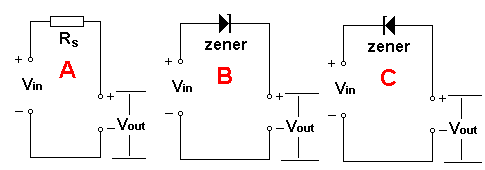Circuit A will produce 5v when say 500mA flows. But if 300mA flows, the voltage will be higher than 5v. If 700mA flows the output voltage will be lower than 5v.
Circuit B has the zener around the wrong way. It will only drop about 0.7v.
Circuit C will deliver 5v if the correct value zener is used and it has the correct wattage-rating.

Here is a mistake from an electronics forum: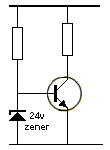The zener diode is supposed to turn the transistor off when the supply is greater than 24v.
I don’t know if the transistor is supposed to be turned ON or OFF, but the circuit will not work.
After reading this article, you will know that a zener is “invisible” until the voltage reaches the zener-value, but the voltage across the base-emitter of a transistor will NEVER rise above 0.7v.
The zener will never see 24v.
The following circuit will work: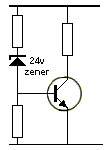When the supply is slightly higher then 24v, the transistor will turn ON.

## THE ZENER ANALOGY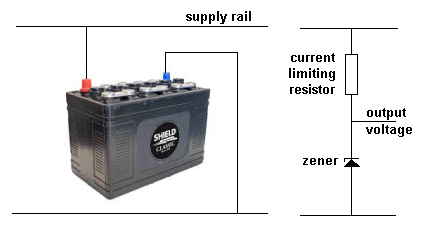A battery and a zener diode behave in a very similar way and if you understand this, you will see why it is very difficult to charge a battery from a power supply. And you understand how a zener diode “eats-up” all the current when the voltage rises over a certain value.

The battery is being charged from the supply rail. We know it will not get charged when the supply rail is less than the voltage of the battery.
As the voltage of the supply rail increases, the battery will take more current but its voltage will not rise above 13.6v.

That’s the way the zener works.
It will not allow any current to flow though it until the supply rail is equal to the value printed on the diode. This is its breakdown voltage.
If you increase the voltage of the supply rail, the voltage on the top of the zener diode will not increase, but the extra current that will flow through the current limiting resistor, will flow through the diode.

Knowing this helps you charge a battery.
If you connect the battery to a power supply and increase the voltage, a point will come when the voltage is equal to the maximum of the battery (13.6v) and if you continue to increase the voltage, a VERY HIGH CURRENT WILL FLOW and maybe damage your power supply.
The technical answer to this is: There is no current limiting resistor. THE LED - How it is like a zener diode !
Now we are going to talk about something AMAZING.
It’s a LED - LIGHT EMITTING DIODE. It is just like the diodes we have described above and just like the ZENER DIODES we have talked about above.
Yes, that’s right a LED is just like a diode and functions just like a diode but it just like a diode with a window so you can see the current flowing through it.
This is a wonderful advantage. Now you can see when a diode or zener diode is WORKING !
But a LED has two slight differences. It is not like a 400v diode but just a 5v diode. A LED can only withstand about 5v to 7v in the reverse direction before it is damaged. This means you can use a LED on any circuit up to about 5v.
And the other thing is a LED can only pass about 20mA, whereas a 1N4004 diode will pass about 1,000mA.
And a LED is just like a zener, but instead of a zener voltage of say 12v, a LED had a zener voltage of 1.7v for red and about 3.6v for white.
Now you can use all your knowledge about diodes and zeners and reference it to a LED in a circuit where less than 20mA is flowing , the voltage is less than 5v and the zener reference is 1.7v to 3.6v or any multiple or addition of these voltages.
This concept is NEW. It has never been explained or covered in any text book in the way we are explaining it.
No-one has thought to show the similarities and that’s why you get so many questions on electronics forums about the operation of a LED and why you need a current-limiting resistor.

## THE TRANSISTOR AS A ZENER DIODE

A transistor exhibits “ZENER CHARACTERISTICS” when placed in a circuit as shown in the following diagram:The base-emitter junction is “reverse biased.” In other words, it has voltage across it that is opposite to the normal way the transistor is used.
The junction cannot withstand a very high voltage in this direction and it BREAKS-DOWN.
In other words it starts to allow current to flow through the junction and a voltage is created across this junction. The resistor is provided to prevent a high current to flow through the junction and to allow the junction to break down and create the natural value across the two leads.
This is called ZENER BREAKDOWN.
The voltage will depend on the type of transistor and can be anywhere from 6v to 8v. For a particular type of transistor it will be quite stable (fixed) and you can allow say about 10mA to flow through the junction. The transistor will not be damaged.

## THE SHUNT REGULATOR

We will now combine all the facts we have learnt from above to produce a circuit called a SHUNT REGULATOR.
A Shunt Regulator takes a high voltage (containing ripple) and produces a lower FIXED voltage with very little ripple.
We have learnt that:

1. A BRIDGE converts AC to DC (pulsating DC. DC with ripple).
2. An electrolytic smoothes the pulsating DC and reduces the ripple.

A shunt regulator FURTHER REDUCES THE RIPPLE and produces A FIXED VOLTAGE.
A SHUNT REGULATOR consists of two components: A resistor (called the current limiting resistor) and a zener diode.

Here’s how the SHUNT REGULATOR works.

Firstly, the zener diode and resistor work just like a dam with an overflow pipe at the top. If the water level in the dam does not reach the pipe, NO water overflows. When the water reaches the pipe, it overflows through the zener pipe. If the water level rises further, more water flows through the zener.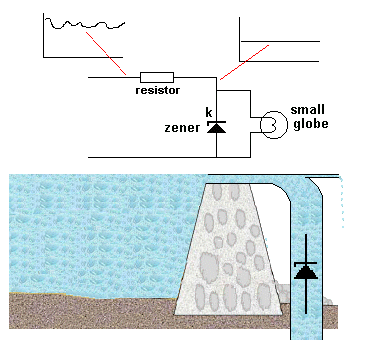The height of the water is never above the small overflow pipe.
The small overflow pipe is connected to the globe and the brightness of the globe is constant because the voltage on the cathode of the zener is fixed (by the action of the zener diode).
It does not matter how much ripple is present in the incoming waveform, the value of the resistor is chosen to eliminate ALL THE RIPPLE.

The mathematics to work out the value of the resistor is very complex as it involves the resistance of the resistor, its wattage and the wattage of zener.
This is covered in the article: The Power Supply and also above with a set of tables.
The height of the small overflow pipe can be taken to a LOAD such as GLOBE.
The load can only be a SMALL LOAD. In other words, it can only require a small current, such as a small torch globe.
If the load takes ALL THE CURRENT (coming from the resistor), the zener will get NO CURRENT and it will DROP OUT OF REGULATION.
In other words, the voltage on the cathode of the zener will drop.
This is exactly like putting a pump on the small overflow pipe and sucking all the water from the zener: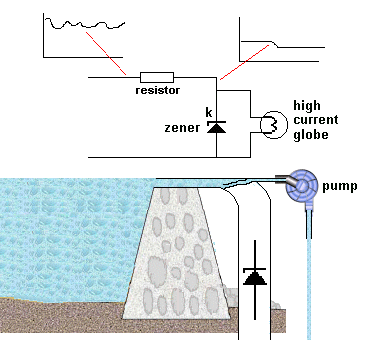Question Why use a SHUNT REGULATOR?

Answer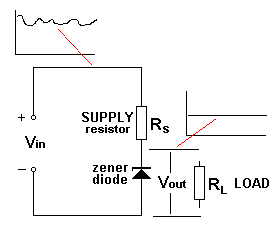A SHUNT REGULATOR is a very cheap and simple way to produce a clean output voltage (with very little ripple) from a higher voltage that has ripple.
You must design the circuit so that the output voltage is a few volts below the lowest dip in the voltage being supplied. The Shunt Regulator circuit is not MAGIC. It simply deliver the voltage below the ripple that has NO RIPPLE !!

We can now add the bridge and smoothing electrolytic: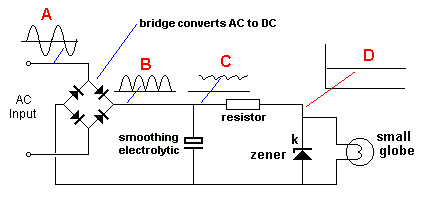Waveform A is an AC voltage and you can see it rises to a peak above the zero-voltage line and equally below the 0v line.
Waveform B represents the waveform as it emerges from the bridge. (The electrolytic is not connected to detect this waveform.)
Waveform C shows the effect of the electrolytic. It stores energy from the peaks and delivers the energy when the waveform drops. The result is DC with a small amount of ripple.
Waveform D is very smooth DC at a voltage determined by the voltage of the zener.

Question In the diagram above, describe the shape of the output voltage D if the electrolytic is removed

Answer The pulsing DC will produce a waveform on the cathode of the zener as shown in the following diagram: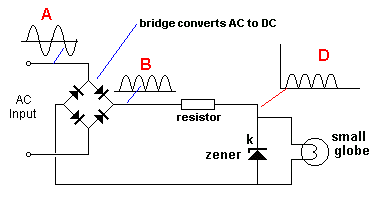This will not matter if the load is a globe but if the load is an amplifier, the power supply will produce HUM.

## ZENER QUESTION

Here is a circuit from the web and an answer from the instructor:
If the voltage across the load tries to fall, then the Zener takes less current. The current through the resistor and the voltage across the resistor both fall. The voltage across the load remains at its correct value.The description is incorrect. Can you see the mistakes????
This circuit is called a CURRENT DEFINED CIRCUIT. In other words, you describe its action when a change in CURRENT occurs.
If the load takes more current, it robs this current from the zener.
When the circuit is working correctly, the ZENER and LOAD are sharing current and the current through the resistor R DOES NOT CHANGE.
You can see how many mistakes are in the answer above:
The current through the resistor DOES NOT CHANGE, the voltage across the resistor DOES NOT CHANGE.
This means the voltage across ALL THE COMPONENTS does not change and it is pointless describing the circuit in terms of voltage.
Now you can see how the electronics engineer that described this circuits does not understand how to describe its operation.
He started with the wrong premise by saying: _If the voltage across the load tries to fall.
_He should have said: . . .due to an increase in current required by the load.
He would then be able to describe the circuit using only CURRENT changes.

## THE SERIES REGULATOR

The SERIES REGULATOR can be identified by the fact that the zener diode does not pass all the current when the load is removed.
This type of circuit is much less wasteful than the Shunt Regulator.
You can also see the current (actually the voltage and the current) passes through the controlling device (the transistor) and then the load, just like two components in SERIES.
In fact, we have a shunt situation with the zener and 1k resistor as the voltage across the 1k is 8v and this causes 8mA to flow through the 1k and thus the zener has 8mA flowing through it.
If the transistor has a gain of 50, it will allow up to 8 x 50 = 400mA to flow through the collector-emitter circuit.
But if you try to take more than 400mA (by connecting a load with a very low resistance) the whole circuit will DROP OUT OF REGULATION.
This means the output voltage will drop below 15.4v.
Let’s see how this happens.
When the load takes 50mA, the collector-emitter leads of the transistor deliver this current and the base takes 1mA from the zener diode. This means the zener sees 7mA.
When the load takes 400mA, the base takes 8mA and the zener diode gets NO current.
Up to this point, the circuit works perfectly.
But if you take 450mA, the base requires 9mA and when 9mA flows through the 1k resistor, the voltage across the resistor is 9v.
This means the base is 24v - 9v = 15v and this voltage is lower than the breakdown voltage of the zener.
The zener only has the capability of preventing the voltage rising above 16v, it does not have any control when the voltage delivered to it is less than 16v.
The output voltage is now 14.4v and the circuit is said to have DROPPED OUT OF REGULATION.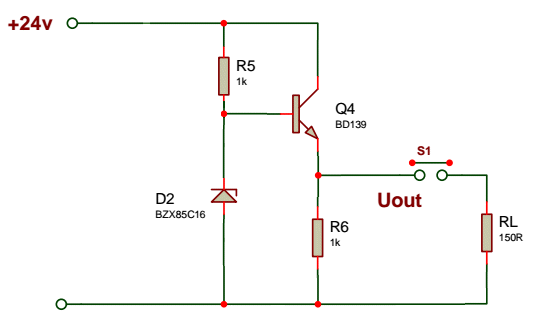Here is an unusual use for a zener diode in the bridge of a power supply: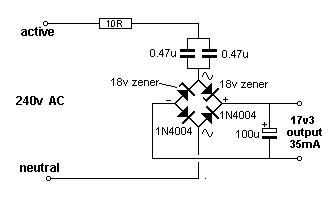This is a very special type of power supply called a TRANSFORMERLESS POWER SUPPLY and it shows the effect of putting a diode in the bridge that has a very low breakdown voltage. Normally the 4 diodes will have a peak reverse voltage (breakdown voltage) of 200v or 400v and this effect will not be noticed. But when two 18v zeners are included to demonstrate the effect of “breakdown voltage” the output of the supply is 18v minus 0.7v across the lower diode.

## CURRENT SHARING

The current though the zener and the current though the small globe is called CURRENT SHARING. They share the current coming from the resistor. The resistor is called a FEED RESISTOR or FEEDER RESISTOR or CURRENT LIMITING RESISTOR or CURRENT DETERMINING RESISTOR. It is NOT a LOAD RESISTOR.
It is designed to deliver CURRENT to the zener and globe. The globe is the LOAD.
A shunt regulator consisting of a zener (and resistor) is designed to deliver a small current. If a large current is required, a transistor is added to the circuit, called a PASS TRANSISTOR or a number of different circuits can be used.

We will now cover CURRENT SHARING: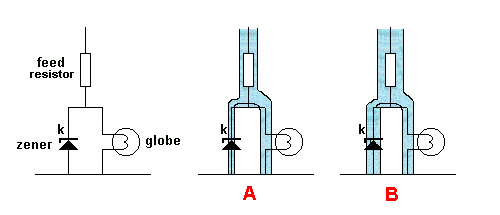Diagram A above shows the correct CURRENT SHARING between the zener and globe. The zener should only be taking a few milliamps as this current is wasted and the zener is only required to provide a fixed voltage.
However if the load is removed, all the current taken by the load will not flow through the zener and that’s why the zener must be capable of dissipating this wattage.
Diagram B shows what will happen if the supply voltage increases. The CURRENT through the feed resistor will increase. This is similar to more water flowing though the pipe containing the feed resistor and the extra water will flow though the zener.
Here is the reason:
The voltage on the cathode of the zener is fixed (the voltage delivered to the globe is fixed). This means the current taken by the globe will remain constant. Thus any extra current can only flow though the zener.

We will now change the globe for a motor: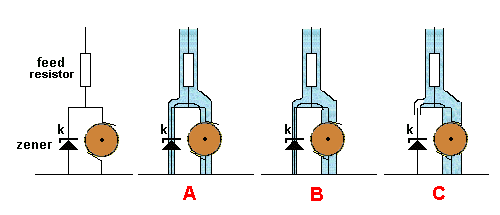If the supply voltage remains constant and the motor takes more current, it robs the current from the zener. This shown in diagram B above. If the motor takes even more current, it will take ALL THE CURRENT from the zener. This shown in diagram C above.
Up to now the voltage across the motor is constant. But if the motor wants more current, the SHUNT REGULATOR will drop out of regulation and the voltage across the motor will DROP.
The motor may or may not get more energy from the supply, but we will not go into this condition because the SHUNT REGULATOR has ceased to perform.

The SHUNT REGULATOR is called a SHUNT REGULATOR because the zener is connected directly across the voltage being delivered to the LOAD and it “shunts” (takes away) ALL the current being delivered by the resistor (if the load is removed). When a LOAD is connected, it takes current from the zener and it can do this until almost all the current is taken.
You must leave a small current through the zener to keep the circuit in REGULATION.
The SHUNT REGULATOR is a very wasteful design as current is flowing ALL THE TIME. If the load is not using the current, it is being wasted through the zener.

CURRENT SHARING can also be also be applied to placing two equal diodes in parallel. This can be ordinary diodes or zener diodes.
Take the case of “power diodes.”
Suppose you have a 1-amp power supply and intend to use four 1N4004 diodes in a bridge.
We have already mentioned that this type of diode has a voltage drop of 0.7v when the current is about 500mA but increases to 1.1v when the current is 1 amp.
By using an extra set of four diodes in parallel with the first set, the current through each diode will be shared. The current may not be shared equally, but it will be much less than 1amp (through each diode) and the overall wattage-loss will be less and this will be shared between two diodes. Overall, a very good outcome.

## ACTUAL ZENER CIRCUIT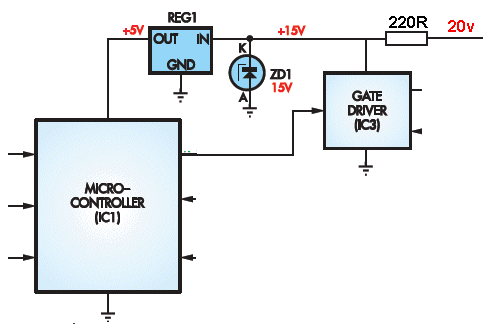Here is a circuit using a 15v zener diode.
Here’s how to look at this circuit:
The input voltage is 20v.
Remove the three “rectangles.”
The zener diode will create a rail voltage of 15v and all the current from the 20v supply will pass through the zener.
This current can be worked out by looking at the 220R resistor. It has 5v across it and the current flowing will be: V = I x R 5 = I x 220 = 5/220 = 22mA
The regulator requires a small current to drive the transistors inside the chip. Say this is 2mA.
The Microcontroller requires a small current to operate. Say this is 6mA.
The gate driver uses 1mA.
The remaining 13mA flows through the zener.
Suppose the microcontroller turns ON and output line and takes an extra 13mA.
This means NO CURRENT will flow through the zener and it will DROP OUT OF REGULATION.
The rail voltage will remain at 15v but the zener will have no part in this circuit (at the moment).
If the micro wants say 2 more milliamp (to drive another output device) the 15v rail will drop slightly.
The 5v rail will remain at exactly 5v and the 15v rail will drop a small amount.
This circuit is called CURRENT SHARING.
The 3 devices in the rectangles SHARE the current with the zener. There is 22mA to share between the 4 items.
You can also say the 3 devices TAKE current from the zener or ROB (steal) current from the zener and when all the 22mA is STOLEN, the 15v rail drops in voltage and we have lost what is called ZENER REGULATION.

## GATING - how a diode can be used as a GATE

The technical way to describe a diode is:
A diode only allows current to pass in one direction. This also means a diode only allows voltage to appear on the cathode when the voltage on the anode is above 0.7v.

We can use this feature to INHIBIT (stop) an oscillator and also produce circuits where two or more inputs determine the output of a circuit.
This is called GATING.
We will cover two features, Gating An Oscillator and creating Diode Gates

## GATING AN OSCILLATOR

We are not interested in how the oscillator works. We want to STOP or INHIBIT the oscillator via an input signal.
The simplest type of circuit to “control” or “gate” is a DIGITAL OSCILLATOR. This is an oscillator made up of a BUILDING BLOCK contained in an INTEGRATED CIRCUIT.
The Building Block is also called a GATE and we have two terms called “GATE” in this discussion.
The Building Block may be an AND gate, OR gate, Inverter or Schmitt Trigger.
The other “gate” in this discussion is the action of turning ON or turning-OFF the oscillator.
The “gate” is the diode on the CONTROL LINE and it is taken HIGH or LOW to control the oscillator.

In the following circuit, the INTEGRATED CIRCUIT (IC) contains 6 Schmitt Triggers and each Schmitt Trigger is called a BUILDING BLOCK or Schmitt Gate. It oscillates due to the resistor R and capacitor C.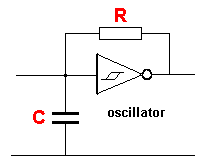The voltage on the capacitor “C” rises to 2/3 rail voltage (via resistor “R”) and this is detected by the IC to make the output LOW. The capacitor now discharges to 1/3 rail voltage via resistor R and the IC detects a LOW to make the output HIGH.

An animation of the circuit is available below:

```<!-- TODO FIXME COLIN: find and add the animation here -->
```

A gating diode can be placed on the “control line” to control (inhibit) the oscillator: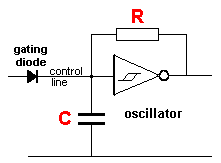When the gating diode is taken HIGH, the oscillator is “jammed” (inhibited - frozen):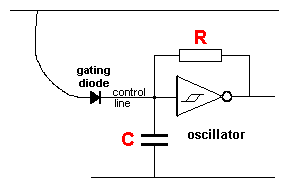The oscillator is INHIBITED

In the diagram above, the voltage through the gating diode will keep the capacitor charged and prevent the IC changing state. The oscillator is INHIBITED.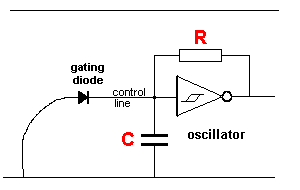The oscillator is NOT inhibited

In the diagram above, the gating diode does NOT inhibit the oscillator. The oscillator produces a square-wave output.

If the gating diode is reversed, a HIGH on the cathode will NOT inhibit the oscillator: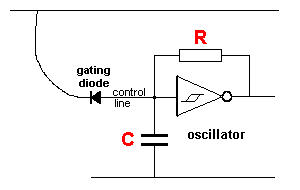The oscillator is NOT inhibited

A gating diode connected to 0v, as shown below, will inhibit the oscillator: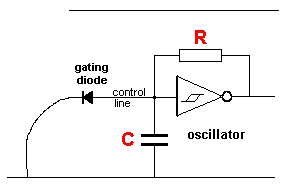The oscillator is INHIBITED

The gating diode will only allow a voltage of 0.7v to appear across its leads. This voltage is too low for the input of the IC and thus the circuit will not change state. The oscillator is INHIBITED.

The gating diode is normally connected to the output of a controlling circuit, as shown below: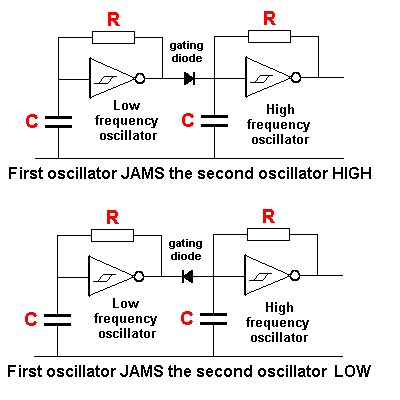The first oscillator controls the second oscillator via the gating diode.
The operation of the Schmitt Trigger oscillators is fully covered on this site - you can search at the top of the page for relevant articles. We are only covering the operation of a diode in this discussion.

## THE GATING DIODE

It is important to understand the effect of a gating diode on the operation of an oscillator (as shown in the diagrams above).

Question Is the oscillator inhibited (stopped - halted - frozen) when the anode of the gating diode is HIGH?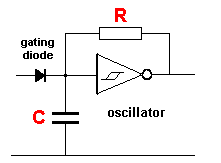Answer Yes. The voltage via the diode will keep the capacitor charged and the oscillator will be frozen with the output low.

Question Is the oscillator inhibited when the cathode of the gating diode is HIGH?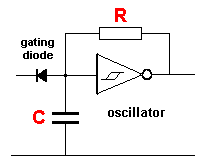Answer No. The voltage on the cathode will not pass through the diode and the operation of the oscillator will not be affected.

Question Is the oscillator inhibited when the cathode of the gating diode is LOW?Answer Yes. The 0v on the cathode will put a maximum of 0.6v on the anode and this will keep the capacitor discharged and the oscillator will be frozen with the output high.

Question Is the oscillator inhibited when the anode of the gating diode is LOW?Answer No. 0v on the anode will not pass through the diode. The diode will allow a voltage of at least 100v to appear on the cathode and thus the operation of the oscillator will not be affected.

## DIODE GATES

Diodes can be connected so that two or more CONTROL LINES determine the state of an output.
This is called GATING and more-specifically DIODE GATING.
The two diode gates are: AND GATE OR GATE.

An AND GATE is HIGH when both inputs are HIGH.
An OR GATE is HIGH when either input is HIGH.

Notes:

1. For a Diode Gate, we are not concerned with the 0.6v to 0.7v drop across the diode.
2. A Diode Gate is classified as DIGITAL GATE. In other words, the output is either HIGH or LOW.
3. When it is HIGH, the output is as close to the rail voltage of the system as possible. This voltage is normally 5v, but it can be 3v3 for a 3v3 system or 12v of other systems.
4. When it is LOW, the output is as close to 0v as possible.

## THE DIODE OR GATE

The voltage and current is passed to the output by the incoming signal and a pull-up resistor is not needed. A pull-down resistor will prevent the output “floating.”
The output will go HIGH when line A OR line B is HIGH (and also when BOTH inputs are HIGH).
A voltage-drop of 0.6v is lost across the diode but this will not affect any circuit using this gate.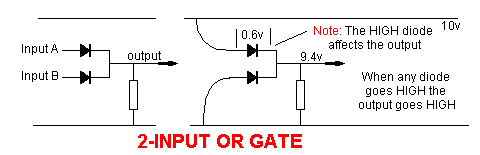## THE DIODE AND GATE

For the diode AND gate, BOTH input A AND input B must be HIGH for the output to be HIGH.
The diode AND gate works in a slightly different way to the diode OR gate.
The pull-up resistor delivers the output voltage and current.
The input lines ALLOW the output voltage to rise when BOTH inputs are HIGH. The output current of the AND gate is determined by the value of resistor R. When the output is low, this current is termed BLEED CURRENT and flows through the diode(s) to the 0v rail. This current is “waste” current and must be kept to a minimum for good design.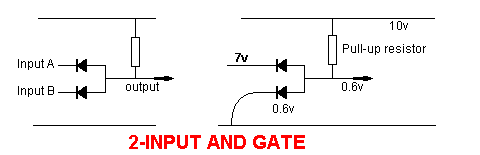Note:
NAND and NOR gates cannot be produced with diodes and resistors as these gates involve INVERSION and a diode cannot provide inversion. A transistor or IC is needed to provide inversion.
The OR and AND gates above are called DIGITAL GATES because the output is HIGH or LOW. This condition is created because the input lines are DIGITAL LINES - they are either HIGH or LOW.

Question Is the following circuit an AND gate or OR gate: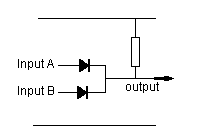Answer The two diodes are connected around the wrong way. The circuit is not and AND gate or OR gate.

Question Is the following circuit an AND gate or OR gate: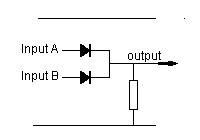Answer The gate is an OR gate.

## THE TRANSISTOR

A transistor can be considered to have a diode between between the base and emitter leads because these two leads behave just like a diode. We have shown an NPN transistor in the diagram below, but the same applies to a PNP transistor.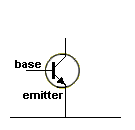An NPN Transistor

This fact is important to know when analysing circuits such as this one: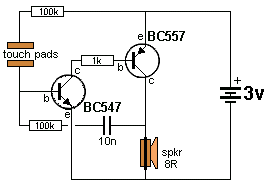Lie Detector

The circuit will not start to turn ON until the voltage on the base of the NPN transistor is 0.6v.
This voltage is provided by your finger on the Touch Pads and the top 100k resistor.

At the beginning of this article we mentioned the fact that the voltage across a diode can be increased from 0v to 0.55v and nothing flows through the diode.
This is exactly the same effect with the base of a transistor.
The transistor does not conduct until the voltage on the base reaches 0.6v and if the voltage is increased, above 0.55v, current will start to flow “through the diode” - “through the base lead to the emitter.”
Although a diode and base-emitter junction of a transistor are NOT the same thing - we can use this simple explanation to understand how the two components work.

In the case of a diode, as the voltage is increased over 0.6v, more current flows through the diode. In the case of the base of a transistor, as the voltage is increased over 0.6v, the transistor turns ON more and more current flows through the collector-emitter terminals.
(The voltage 0.6v can range from 0.6v to 0.9v, depending on the type of diode and the current flowing.)
The whole point to understand is this: NO current flows until the voltage across the diode is 0.55v and as the voltage is increased, more current will flow (in the case of a transistor).
In the case of a diode, you can add this interpretation: As the circuit requires more current, the additional current will flow though the diode and create a higher voltage-drop across it.

## BREAKDOWN

When a diode, zener diode and/or transistor sees a HIGH VOLTAGE across ANY of the leads, it will BREAKDOWN.
No semiconductor device can withstand a very high voltage and we will look at what happens.
This is a very important topic because we use this breakdown feature in some circuits and other times we need to know how to prevent breakdown.
If a transistors sees a high voltage such as a spike (and the voltage has a reasonable amount of current) the transistor will be instantly damaged.
If the current is low, the transistor can be repeatedly operated with the feature.
Here’s what will happen to a diode, zener diode and transistor: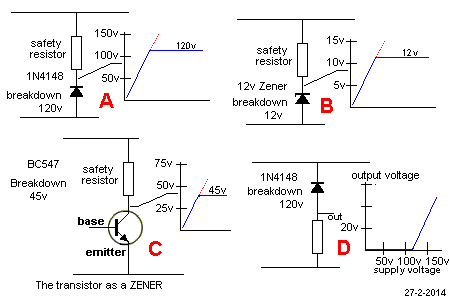The voltage across a signal diode or power diode or zener diode will be equal to rail voltage when the voltage is below the breakdown or Avalanche voltage of the diode. In other words, no current will flow through the safety resistor and no voltage will be dropped across this resistor.
However, when the voltage reaches the Peak Inverse Voltage for a Diode, or the avalanche voltage for a zener, the device will breakdown and cause current to flow through the device so that the voltage on the cathode will not rise any further. As you increase the voltage, the current through the device will increase but the voltage across it will not change. The device will get hotter and hotter until it fails with overheating.

The same will occur with a transistor. When the voltage on the collector reaches the maximum for the transistor, it will breakdown and the voltage across the collector-emitter will remain at this voltage.

Fig D shows how a diode (or zener diode) can be used to pass voltages that are higher than the breakdown voltage of the device. Nothing flows though the device until the breakdown voltage is reached and then the exact-same-waveform flows though the device.

Note: When the three devices are connected in the reverse direction the diode and zener will drop (breakdown) at 0.6v and the transistor will breakdown at a very low voltage.

## WHY DOES A DIODE FAIL?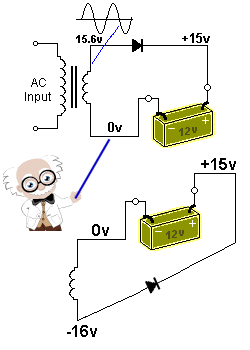The PIV on the diode is 31v

Here we have a simple circuit for charging a battery. The diode is 4 amp with a PIV rating of 30v. The PIV rating is the voltage the diode is capable of withstanding in the opposite direction. This is just an example of what will happen.
When the output of the transformer is positive on the top, the voltage across the diode is about 0.6v (or as high as 1v when 4 amps flows) and the wattage dissipated by the diode is about 4 watts.
When the voltage reverses direction, the top output of the transformer becomes NEGATIVE and it just like the winding “flips-over” to produce the second circuit.
The diode sees a negative voltage on one end and a positive voltage on the other lead.
This means a total of 15 and 16 = 31 volts is across the diode.
When the voltage reaches 30v, this is the PIV voltage for the diode (this can also be called the zener voltage) and it BREAKS DOWN at 30v so that any voltage higher than 30v allows current to flow through the diode FROM THE BATTERY to the TRANSFORMER. This current will be very large because the battery is capable of delivering a high current and the transformer winding will be a thick wire and if it is capable of delivering 4 amps when charging the battery, it will accept a higher current in this “break-down” condition.
The current will be as high as 5 to 10 amps or more.
Suppose 5 amps flows.
The wattage dissipated by the diode will be 5 x 30 = 150 watts. Obviously the diode will be destroyed very quickly.
That’s why a diode in a 12v battery-charger needs to have a PIV rating higher than 30v.

## HEATSINKING

You might not think a power diode needs to be HEATSINKED because there are no heat-fins on a diode. But the leads and pads on the PC board form a very important part of getting rid of the heat generated.
The author has seen examples of one pad being smaller than the other and the lead heated up the solder to produce a dry joint.
In another case, the small pads resulted in the diode overheating and “shorting;” and other diode “going open.”
So, the quality of the heatsink is VERY IMPORTANT.
If you cannot hold your finger on a diode for 10 seconds, it is TOO HOT.
Adding extra-wide pads and tracks on the underside of the board is a very good idea.
Power diodes are very robust, however it is good engineering to add extra track-work to prevent over-heating.

I am working on an automotive air-conditioner system that keeps blowing fuses.
I disconnected the compressor and the fuse still blows. I figure I have either a short to ground on the wire from the relay to compressor…or maybe there is something going on with the diode.
Here is the circuit: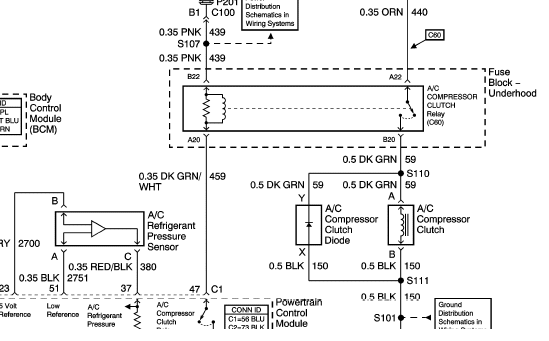Circuits with lots of plugs and numbers and figures are very hard to follow.
Here is a simplified circuit to show the diode is connected between the positive line into the compressor clutch and ground: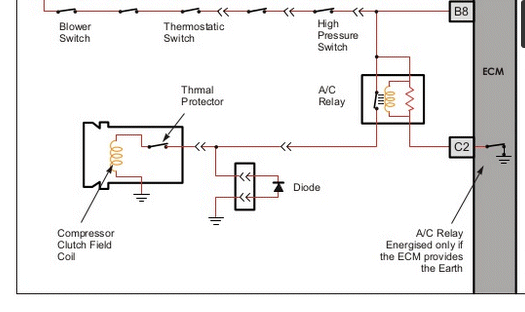If the diode is “shorted” it will put a low resistance on the line into the clutch and “blow the fuse.” This is the first component to replace, as a short-circuit in the wiring is a rare problem.
Remove the diode and check the resistance in both directions with a multimeter set to LOW OHMs. Alternatively, connect the diode to the positive lead of a 12v globe and then a 12v battery. Now turn the diode around the other way. The globe should NOT illuminate.
A diode can fail due to vibration, heat, stress on the leads, or voltage spikes. Although these faults are rare, you must remember these are all possibilities as well as the diode going intermittent and failing when a high current is passing through it.
You must remember also, a diode is made of materials that break-apart very easily. The only thing that keeps them together is the body of the diode. If the body of the diode expands, the junction separates.

Now go to:

DIODE TEST

How a Capacitor Works

You will find a lot of helpful material on “electronics in general” on these pages: Spot Mistakes

Now go to Basic Electronics 1A: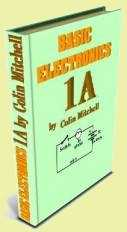electronics
writing
PIC-Chips